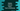# How to remove items from a dictionary in Python## How to remove items from a dictionary in Python:

Python dictionary provides a couple of methods to remove single or multiple items from a dictionary. The dictionaries are used to store key-value pairs in Python. Each value is associated with one key. We can use a key to access the value associated with that key.

Let’s take a look at the below dictionary:

``````given_dict = {1: 'one', 2: 'two', 3: 'three'}

print(given_dict)``````

Here, we initialized a dictionary given_dict and print the content of the dictionary. It will give the below output:

``{1: 'one', 2: 'two', 3: 'three'}``

It is a simple program. It is printing the content of the dictionary.

To delete these items, we have four different methods, del, pop, popItem and clear. Let’s learn how these works with example for each:

### del:

The del keyword can be used to remove a specific pair from a dictionary or we can delete the complete dictionary.

The following example removes a specific key-value pair from a dictionary:

``````given_dict = {'one': 'Jan', 'two': 'Feb', 'three': 'March'}

del given_dict['three']

print(given_dict)``````

It will remove the pair `'three': 'March'` from the dictionary.

``{'one': 'Jan', 'two': 'Feb'}``

You can also delete the complete dictionary variable:

``````given_dict = {'one': 'Jan', 'two': 'Feb', 'three': 'March'}

del given_dict

print(given_dict)``````

Since the dictionary is deleted and we are trying to print it after it got deleted, it will throw one error.

``NameError: name 'given_dict' is not defined``

### pop:

The pop method takes the key value as its parameter and removes that key-value pair from the dictionary. This method also returns the value of that key. For example,

``````given_dict = {'one': 'Jan', 'two': 'Feb', 'three': 'March'}

print(given_dict.pop('three'))

print(given_dict)``````

I am also printing the return value of pop in this example. The first print statement prints the return value of pop and the second print statement prints the final dictionary.

``````March
{'one': 'Jan', 'two': 'Feb'}``````

### popitem():

The popitem method doesn’t take any argument. It removes the last inserted pairs from a dictionary and returns a tuple. The tuple holds both key and value. Let me modify the previous example:

``````given_dict = {'one': 'Jan', 'two': 'Feb', 'three': 'March'}

print(given_dict.popitem())

print(given_dict)``````

It will print:

``````('three', 'March')
{'one': 'Jan', 'two': 'Feb'}``````

The clear method removes all elements of a dictionary. The difference between del and clear() is that clear() doesn’t delete the dictionary variable. It empties the dictionary items.

``````given_dict = {'one': 'Jan', 'two': 'Feb', 'three': 'March'}

given_dict.clear()

print(given_dict)``````

It will print an empty dictionary.

``{}``